### Day 16 - Tangent Line - 09.08.15

Update
• New Seats!
• Remediation Procedure
• Unit 1 Test
• Average = 86%
• Questions

Bell Ringer

1. What is a secant line?

1. the instantaneous rate of change

2. the average rate of change

3. the slope of a line

4. the equation of a line

5. straight line joining two points of a graphed function

2. What is a tangent line?

1. the instantaneous rate of change

2. the average rate of change

3. the slope of a line

4. the equation of a line

5. straight line based on the slope at one point

3. Find the slope of the line based on these two points: (0,1) and (3,-4).

1. 5/3

2. 3/5

3. -3/5

4. -5/3

5. none of the above

4. Find the equation of the line based on these two points: (0,1) and (3,-4).

1.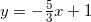2.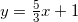3.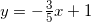4.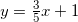5. none of the above

5. If the slope of a line is 4 and a point on the line is (4,-3), what is the equation of the line?

1.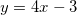2.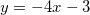3.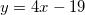4.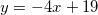5. none of the above

Review
• Pre-calculus
• Slope
• Equation of a Line
• Secant Line vs. Tangent Line (video)

Lesson

Exit Ticket
• Posted on the board at the end of the block.

Homework
• WNQ the following:

Lesson Objectives
• How can the slope of one point be found?

#### In-Class Help Requests

Standard(s)
• APC.5
• Investigate derivatives presented in graphic, numerical, and analytic contexts and the relationship between continuity and differentiability.
• The derivative will be defined as the limit of the difference quotient and interpreted as an instantaneous rate of change.
• APC.6
• ​The student will investigate the derivative at a point on a curve.
• Includes:
• finding the slope of a curve at a point, including points at which the tangent is vertical and points at which there are no tangents
• using local linear approximation to find the slope of a tangent line to a curve at the point
• ​defining instantaneous rate of change as the limit of average rate of change
• approximating rate of change from graphs and tables of values.